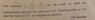# Iterative root finding for the cube root of 17

• AN630078
AN630078
Homework Statement
Hello, I have found this problem below but I am not sure how to approach it, perhaps because I am confused by what I am being asked.
I have attached the question below but I will type it out also (apologies for not writing in LaTEX).

The formula xr+1 =1/2(xr+N/xr^2) can be used to find an approximate value for the cube root of N.
Starting with x0=2 find the value of the cube root 17 to 3.s.f.
Relevant Equations
xr+1 =1/2(xr+N/xr^2)
Firstly, the cube root of 17 is 2.571281591 which is 2.57 to 3.s.f.
Initially, I thought about just approaching this problem using the Newton-Raphson Method when x0=2. In which case; x^3=17
x^3-17=0
Using the Newton-Raphson iterative formula xn+1=xr-f(xn)/f’(xn)
f(x)=x^3-17
f’(x)=3x^2
x0+1=2-(2^3-17)/(3(2)^2)
x1=2.75
x1+1=2.75-(2.75^3-17)/(3(2.75)^2)
x2=2.58264...
x2+1= 2.58264-(2.58264^3-17)/3(2.58264)^2
x3=2.571331512
x4=2.571281592
x5=2.571281591
x6= 2.571281591

With 5 iterations the sequence converges to the the cube root of 17 which is equal to 2.571281591

Should I actually be using the formula from the question, taking N = 17?

In which case;

xr+1 =1/2(xr+N/xr^2)
x0+1 =1/2(2+17/2^2)
x1=3.125
x1+1=1/2(3.125+17/3.125^2)
x2=2.4329
x3=2.652502803
x4=2.53436347
x5=2.590551248
x6=2.561861233
x7=2.576043793
x8=2.568913687
x9=2.572468818= 2.57 to 3.s.f

Therefore, x9 is the first iterate that equals the cube root of 17 when both are given to three significant figures.

Would this be correct? I do not know whether my method is appropriate or if my workings/solution satisfy the question fully? I would be very grateful for any advice 👍

#### Attachments

•32 KB · Views: 226
•Homework Helper
Gold Member
I'm going to write your formula with Latex:
##x_{r+1}=\frac{1}{2}(x_r+\frac{N}{x_r^2}) ##.
This makes sense because ##x=\frac{N}{x^2} ##.It would also make sense because we also have ## x =\frac{1}{2}(x+\frac{N}{x^2}) ##.
I'll leave it for others to comment on this, now that it should be more readable.
One simple comment is you will find different iterative expressions converge at different rates. The Newton-Raphson method converges much quicker.

Last edited:
•Delta2 and sysprog
Homework Helper
Gold Member
additional comment: Even though the Newton-Raphson method comes from derivatives, you can also see how the formula works by looking at the difference of cubes: ## x_{no}^3-x_n^3=(x_{no}-x_n)(x_{no}^2+x_{no}x_n+x_n^2) \approx (x_{no}-x_n)(3 x_n^2) ##. This then gives ##x_{no}=x_n-\frac{x_n^3-x_{no}^3}{3 x_n^2} ##, where ## x_{no} ## is the exact answer. Note ## x_{no}^3=N ##.

•Delta2 and sysprog
Homework Helper
Gold Member
One additional comment: It is a very good job that you did of solving the problem. You compared two different iterative methods, which is perhaps even worthy of extra credit. Your results look very accurate.